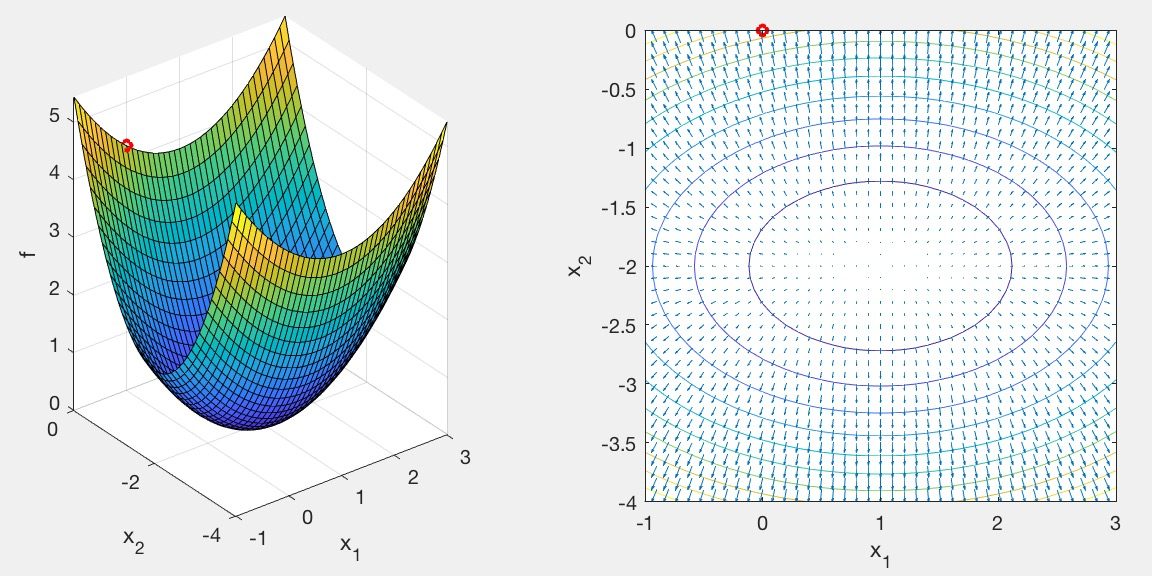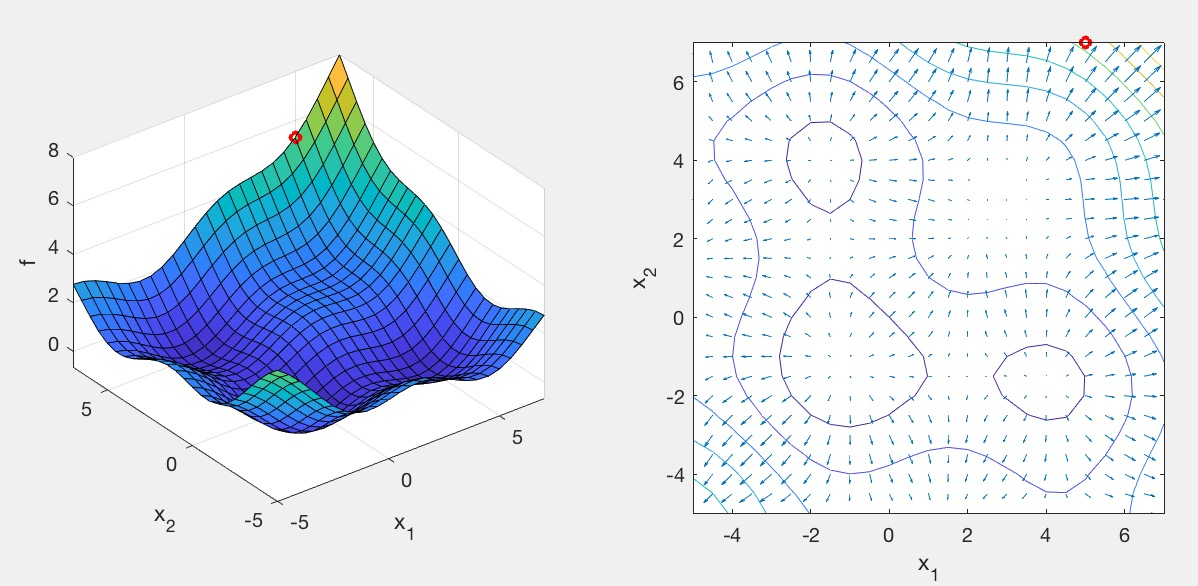# Assignment 4 - Gradient Descent & Newton's Method

Due Wednesday 10/18 at 11:59 pm. You must work individually.

## Overview

In this assignment, you are going to implement and analyze the following optimization algorithms:

• Gradient Descent with Momentum
• Newton's Method

## Base Code

Start with the base Matlab code. Run the code by typing the following at the Matlab prompt:

>> driver(0)The left subplot is a 3D function, f(x,y), that you're trying to find the minimum of. The right subplot is the corresponding contour/quiver plot. The starting point of the search is shown as a red circle.

Currently, two test functions are implemented in fun.m. To switch between different test scenarios, change the driver argument. driver(1) and driver(2) use the same prob, but have different starting points---one is far from the optimum solution and the other one is closer.

Implement the gradient descent algorithm:

• While not converged
• Compute the gradient \vec{g} at current point \vec{x}
• Compute step: \Delta \vec{x} = - \alpha \vec{g}
• Update position: \vec{x} = \vec{x} + \Delta \vec{x}

The test functions in fun.m return the function value f and gradient g, given an input point x. It also computes the Hessian matrix H, which will be used later for Newton's Method. In your driver, pfun(x) has been mapped to evaluate the function without entering fnum, and so these two are equivalent and can be used interchangeably:

[f,g,H] = pfun(x);
[f,g,H] = fun(x,fnum);

To check for convergence, test whether the norm of the current step vector (\|\Delta \vec{x}\|) is smaller than thresh.

Inside the iteration loop, plot the value of x in red in both the left and right subplots. This can be done by copying and pasting the code from lines above the loop:

subplot(1,2,1);
plot3(x(1),x(2),f,'ro:','LineWidth',2,'MarkerSize',5);
subplot(1,2,2);
plot(x(1),x(2),'ro:','LineWidth',2,'MarkerSize',5);

With the current value of \alpha, the convergence is really slow because the iterates keep on overshooting to the other side of the valley. Do some "hyper parameter optimization." In other words, tweak \alpha so that the algorithm converges faster.

## Gradient Descent with Momentum

Try the other test case by running driver(1).This test case has multiple local minima as well as a saddle. If you run your current Gradient Descent code, you will find that it converges to a saddle. We can improve the algorithm by adding momentum:

• While not converged
• Compute the gradient \vec{g} at current point \vec{x}
• Update velocity: \vec{v} = \beta \vec{v} - \alpha \vec{g}
• Compute step: \Delta \vec{x} = \vec{v}
• Update position: \vec{x} = \vec{x} + \Delta \vec{x}

Like before, plot the value of x inside the iteration loop, this time in green. Once the algorithm is implemented, tweak \beta so that the algorithm converges faster.

## Newton's Method

Try running driver(2). This is the same function as driver(1), but zoomed in near the solution. Even though we are starting the search very close to the solution, Gradient Descent, even with momentum, is quite slow to converge. Newton's Method addresses this:

• While not converged
• Compute the gradient \vec{g} and Hessian H at current point \vec{x}
• Compute step: \Delta \vec{x} = -H^{-1} \vec{g}
• Update position: \vec{x} = \vec{x} + \Delta \vec{x}

Like before, plot the value of x inside the iteration loop, this time in blue. Notice how quickly it converges, given that we are close to the solution.

## Report

Please provide a report with your submission (PDF). The report should include the following.

• Short description (number of iterations, parameter values, etc.) along with appropriate figures for:
1. driver(0):
• Gradient Descent: show what happens with \alpha=1.0 as well as with your optimized value of \alpha.
• Momentum: show what happens with your optimized value of \beta.
• Newton: Did it work? Why or why not?
2. driver(1):
• Gradient Descent: show what happens with your optimized value of \alpha.
• Momentum: show what happens with your optimized value of \beta.
• Newton: Did it work? Why or why not?
3. driver(2):
• Gradient Descent: show what happens with your optimized value of \alpha.
• Momentum: show what happens with your optimized value of \beta.
• Newton: Did it work? Why or why not?
4. Create your own driver(3) and driver(4) with a new custom function in fun.m.
• These should use the same function (fnum = 2).
• Make sure to test your derivatives by finite differencing or with Matlab's Symbolic Math Toolbox.
• driver(3) should start the search far from the solution.
• driver(4) should start the search close to the solution.
• Repeat the three questions from (2) and (3) above.
• Do you have any comments about this assignment that you would like to share?

## Evaluation

• For a grade of B, you must implement and analyze both versions of Gradient Descent, and submit a report.
• For a grade of A, you must also implement and analyze Newton's Method.

## What to hand in

Create a zip file named NETID.zip (your email ID; e.g., sueda.zip) so that when you extract it, it creates a folder called NETID/ (e.g., sueda/) that has (ONLY) the following in it:

• Your PDF report
• Your Matlab files

Generated on Wed Oct 11 15:18:39 CDT 2017